# The VARMAX Procedure

### Causality Testing

The following statements use the CAUSAL statement to compute the Granger causality test for a VAR(1) model. For the Granger causality tests, the autoregressive order should be defined by the P= option in the MODEL statement. The variable groups are defined in the MODEL statement as well. Regardless of whether the variables specified in the GROUP1= and GROUP2= options are designated as dependent or exogenous (independent) variables in the MODEL statement, the CAUSAL statement fits the VAR(p) model by considering the variables in the two groups as dependent variables.

```/*--- Causality Testing ---*/

proc varmax data=grunfeld;
model y1-y3 = x1 x2 / p=1 noprint;
causal group1=(x1) group2=(y1-y3);
causal group1=(y3) group2=(y1 y2);
run;
```

The output in Figure 35.24 is associated with the CAUSAL statement. The first CAUSAL statement fits the VAR(1) model by using the variables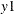,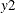,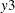, and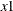. The second CAUSAL statement fits the VAR(1) model by using the variables,, and.

Figure 35.24: CAUSAL Statement Results

The VARMAX Procedure

Granger-Causality Wald Test
Test DF Chi-Square Pr > ChiSq
1 3 2.40 0.4946
2 2 262.88 <.0001

Test 1: Group 1 Variables: x1 y1 y2 y3

Test 2: Group 1 Variables: y3 y1 y2

The null hypothesis of the Granger causality test is that GROUP1 is influenced only by itself, and not by GROUP2.

The first column in the output is the index corresponding to each CAUSAL statement. The output shows that you cannot reject thatis influenced by itself and not by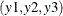at the 0.05 significance level for Test 1. You can reject thatis influenced by itself and not by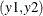for Test 2. See the section VAR and VARX Modeling for details.